A system goes from A to B via two processes I and II as shown in figure. If ΔUI and ΔUII are the changes in internal energies in the processes I and II respectively, then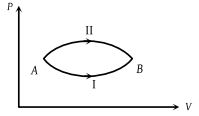(1) ΔUII > ΔUI

(2) ΔUII < ΔUI

(3) ΔUI = ΔUII

(4) Relation between ΔUI and ΔUII can not be determined

Concept Questions :-

Basic terms
High Yielding Test Series + Question Bank - NEET 2020

Difficulty Level:

A thermodynamic system is taken through the cycle PQRSP process. The net work done by the system is -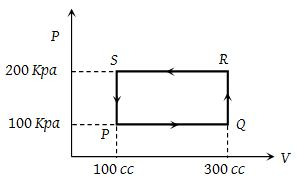(1) 20 J

(2) – 20 J

(3) 400 J

(4) – 374 J

Concept Questions :-

Cyclic process
High Yielding Test Series + Question Bank - NEET 2020

Difficulty Level:

An ideal gas is taken around ABCA as shown in the above P-V diagram. The work done during a cycle is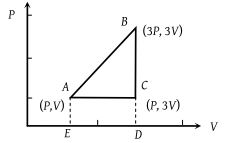(1) 2PV

(2) PV

(3) 1/2PV

(4) Zero

Concept Questions :-

Cyclic process
High Yielding Test Series + Question Bank - NEET 2020

Difficulty Level:

The P-V diagram shows seven curved paths (connected by vertical paths) that can be followed by a gas. Which two of them should be parts of a closed cycle if the net work done by the gas is to be at its maximum value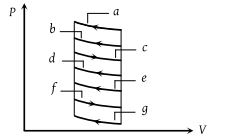(1) ac

(2) cg

(3) af

(4) cd

Concept Questions :-

Work done by gas
High Yielding Test Series + Question Bank - NEET 2020

Difficulty Level:

An ideal gas of mass m in a state A goes to another state B via three different processes as shown in figure. If Q1, Q2 and Q3 denote the heat absorbed by the gas along the three paths, then -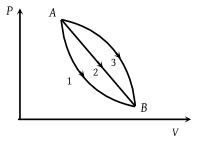(1) Q1 < Q2 < Q3

(2) Q1 < Q2 = Q3

(3) Q1 = Q2 > Q3

(4) Q1 > Q2 > Q3

Concept Questions :-

First law of thermodynamics
High Yielding Test Series + Question Bank - NEET 2020

Difficulty Level:

Which of the following graphs correctly represents the variation of $\beta =-\left(dV/dP\right)/V$ with P for an ideal gas at constant temperature

(1)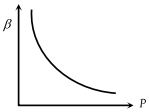(2)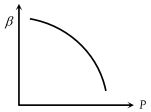(3)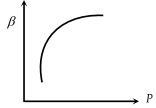(4)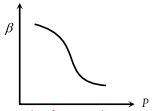Concept Questions :-

Types of processes
High Yielding Test Series + Question Bank - NEET 2020

Difficulty Level:

A thermodynamic process is shown in the figure. The pressures and volumes corresponding to some points in the figure are : ${P}_{A}=3×{10}^{4}Pa,\text{\hspace{0.17em}}{P}_{B}=8×{10}^{4}Pa$ and ${V}_{A}=2×{10}^{-3}{m}^{3},\text{\hspace{0.17em}}{V}_{D}=5×{10}^{-3}{m}^{3}$. In process AB, 600 J of heat is added to the system and in process BC, 200 J of heat is added to the system. The change in internal energy of the system in process AC would be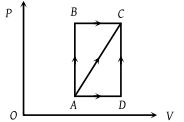(1) 560 J

(2) 800 J

(3) 600 J

(4) 640 J

Concept Questions :-

First law of thermodynamics
High Yielding Test Series + Question Bank - NEET 2020

Difficulty Level:

P-V plots for two gases during adiabatic process are shown in the figure. Plots 1 and 2 should correspond respectively to -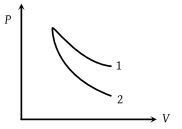(1) He and O2

(2) O2 and He

(3) He and Ar

(4) O2 and N2

Concept Questions :-

Types of processes
High Yielding Test Series + Question Bank - NEET 2020

Difficulty Level:

Four curves A, B, C and D are drawn in the adjoining figure for a given amount of gas. The curves which represent adiabatic and isothermal changes are -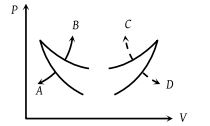(1) C and D respectively

(2) D and C respectively

(3) A and B respectively

(4) B and A respectively

Concept Questions :-

Types of processes
High Yielding Test Series + Question Bank - NEET 2020

Difficulty Level:

In pressure-volume diagram given below, the isochoric, isothermal, and isobaric parts respectively are -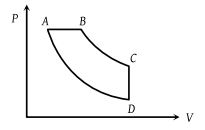(2) DC, CB, DA

(3) AB, BC, CD

(4) CD, DA, AB

Concept Questions :-

Types of processes